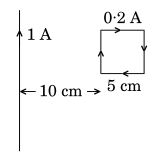# (a) Derive the expression for the magnetic energy stored in an inductor when a current I develops in it. Hence, obtain the expression for the magnetic energy density.(b) A square loop of sides 5 cm. carrying a current of 0.2A. in the clockwise direction is placed at a distance of 10 cm from an infinitly long wire carrying current of 1A as shown. Calculate (i) the resultant magnetic force, and (ii) the torque, if any, acting on the loop.(a) When the external souce supplies current to the inductor , emf is induced in it due to self induction. So, the external supply has to do work to establish current . The amount of work done is:such that,Therefore,The energy density is equal to energy/volume.

Hence,Consider the inductor in the form of a solinoid

thenTherefore(b) We have given,andNow, the force of attraction experienced by length (sp) of the loop is (per unit length).the force is attractive

Now,the force is repulsive.

So, the net force experienced by the loop is (per unit length).The total force experienced by the loop is given as:the resultant magnetic force is attractive in nature

The lines of action of forces coincide, that's why the torque is zero.

### Preparation Products

##### Knockout NEET May 2021 (One Month)

An exhaustive E-learning program for the complete preparation of NEET..

₹ 14000/- ₹ 6999/-
##### Foundation 2021 Class 10th Maths

Master Maths with "Foundation course for class 10th" -AI Enabled Personalized Coaching -200+ Video lectures -Chapter-wise tests.

₹ 350/- ₹ 112/-
##### Knockout JEE Main April 2021 (One Month)

An exhaustive E-learning program for the complete preparation of JEE Main..

₹ 14000/- ₹ 6999/-
##### Knockout NEET May 2021

An exhaustive E-learning program for the complete preparation of NEET..

₹ 22999/- ₹ 14999/-